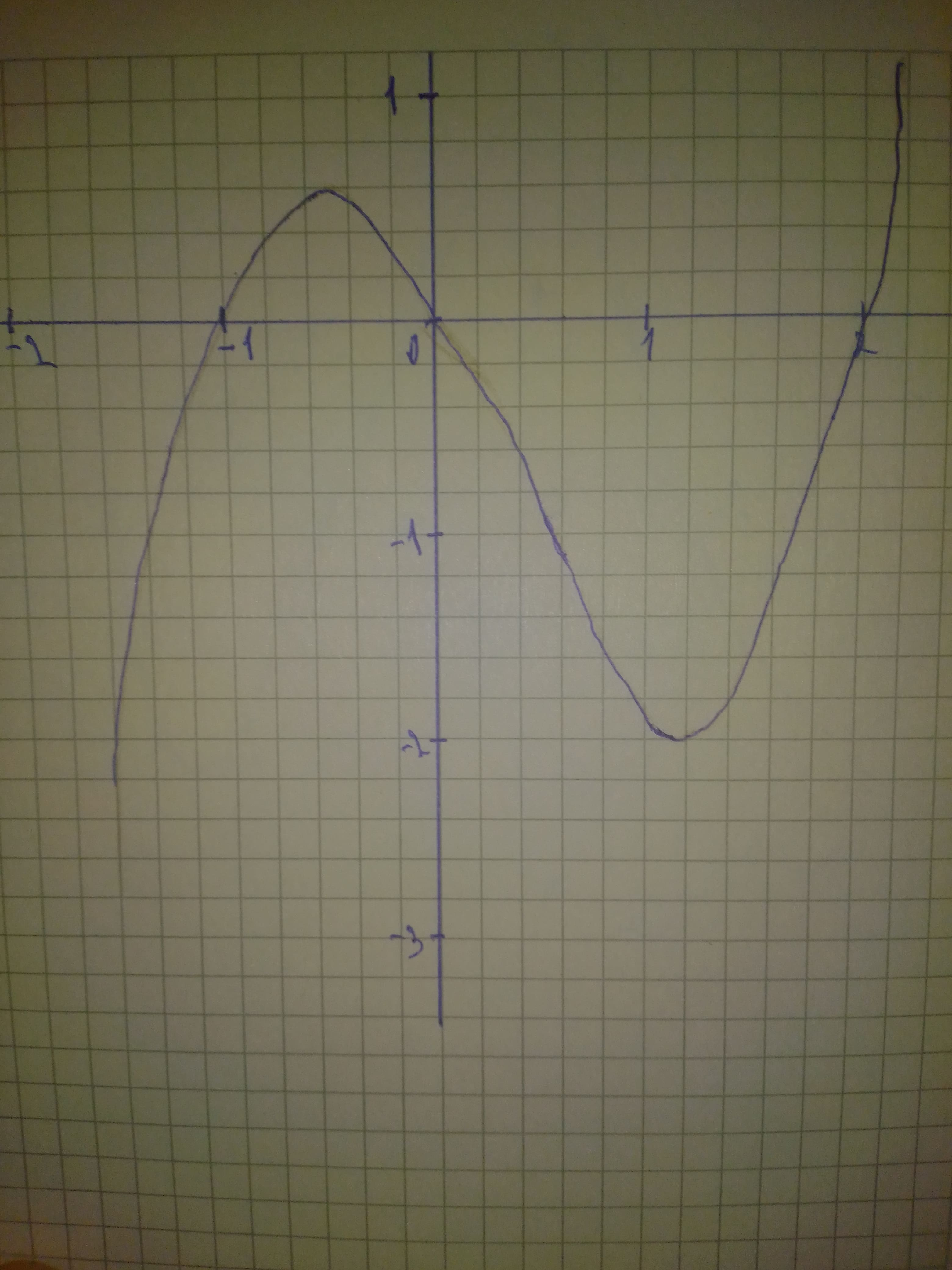# Solve the polynomial inequality graphically. x^{3}-x^{2}-2xgeq0ossidianaZ 2021-01-28 Answered
Solve the polynomial inequality graphically. ${x}^{3}-{x}^{2}-2x\ge 0$
You can still ask an expert for help

• Questions are typically answered in as fast as 30 minutes

Solve your problem for the price of one coffee

• Math expert for every subject
• Pay only if we can solve itAyesha Gomez

We graph the left-hand side of the inequality and find that the x-intercepts are $x=-1,x=0,x=2$:We find the intervals in the graph where $y\ge 0$. The solution to the inequality is $x\in \left[-1,0\right]\cup \left[2,\mathrm{\infty }\right)$